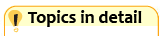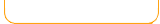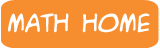Factors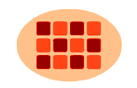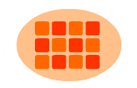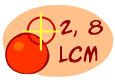Multiples Frenzy
2,3,4,5,6,7,8,9,10,11,12
Multiplication Station
Easy
Multiplication Station
Hard
Greatest Common Factor Least Common Multiple Prime Numbers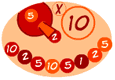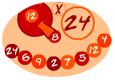Math Lines Math Lines Math Lines Math Lines Math Lines
Add to 10 Add to 20 Add to 24 Add to 50 Add to 60

 What is a factor? Factors are numbers that are multiplied together to get another number. When you factor a number, you are taking the numbers apart and finding the indiviual numbers (factors) that are multiplied within it. Example: What are the factors of the number 6? They are 2 and 3, because 2 x 3 = 6. Also, factors of 6 are 6 and 1, because 6 x 1 = 6. Note: All numbers have the factor of 1, because 1 multiplied by a number equals the same number. So, the factors of 6 are: 1, 2, 3, 6. Example: What are the factors of the number 12? What numbers can be multiplied to get 12? 3 x 4 = 12 2 x 6 = 12 1 x 12 - 12 So the factors of 12 are 1, 2, 3, 4, 6, 12 Example: What are the factors for the number 16? 1 x 16 = 16 2 x 8 = 16 4 x 4 = 16 So the factors of 16 are 1, 2, 4, 8, 16 Prime Numbers A prime number has exactly two factors - the number 1 and itself. It does not have any other factors. Here are some example of prime numbers: Example: the number 7 The only numbers that can divide evenly into 7 are 1 and 7. So 7 is a prime number. Other prime numbers: 2,3,5,7,11,13,17,19,23 ... and the list goes on.

 "Sheppard offers everything from early math to pre-algebra. The lessons include interactive activities to practice concepts. Students can shoot fruit, pop balloons, and even play math man (the math version of pac man!). Fractions, place value, money, and basic operations are some of the areas that are covered. Check it out at http://www.sheppardsoftware.com/math.htm. " --Shannon Jakeman , sjakeman.blogspot.com "Online math games, like the ones that you'll find for free at Sheppard Software, provide a valuable opportunity for children to learn a great deal while they're having fun. The games at Sheppard Software are well written, interactive and very fun to play. It can be very difficult for parents to find productive and worthwhile activities for children on the Internet; however fun online math games do offer a wonderful alternative. This free section of Sheppard Software was written for children. Mostly all of the material you'll find at the site is completely free for kids to use. These fun online math games cover all of the math fundamentals, including early math, basic and mixed operations, fractions, pre-algebra, dollars & cents and place value. While teachers often use worksheets to solidify the math concepts learned in the classroom, these sort of games actually have the same educational impact on the child, and the format of the examples as an exciting game keeps the child engaged, interested and coming back for more. From the main page parents or children will find a simple and easy to navigate menu featuring the different levels of math games and the various math concepts that are available. Children from pre-k up to 8th grade will find fun online math games that teach important math skills that are appropriate for their age level. Sheppard Software offers a couple of cute games for the youngest math students. In this game, called Bugabaloo Addition, children are shown a number of "bug shoes" on the left and the right. The game asks the child to add the two groups of shoes together, and then pick the bug with the correct number printed on it. This game teaches addition through a graphical display that lets the child see and count the shoes. With the numbers right underneath, children easily make the association between the visual cues and the act of addition. For slightly older kids, there are a number of very popular arcade-style "popup" math games. In these games, the child is presented with a math problem and must find the creature that's holding the correct answer and smack it on the head with the hammer. The comical expression the creature makes and the sound effects make this game so fun that children will forget that they're learning math! " --Written March 30, 2010 by Ryan Dube in Educational Freeware -- Reviews of the best learning games, software, and websites --http://www.educational-freeware.com/online/sheppard-math.aspx